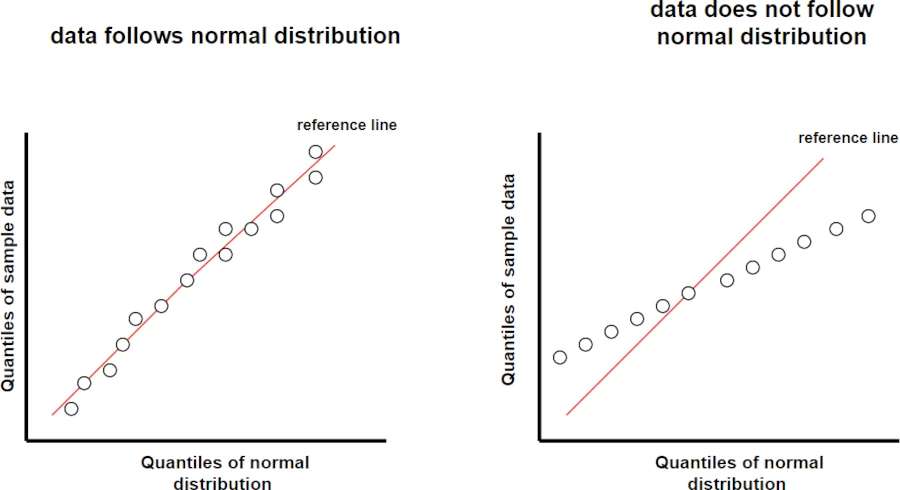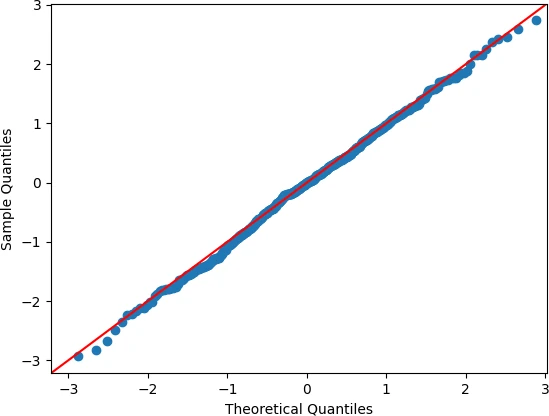# Q-Q plot in Python

The Q-Q plot (Quantile-Quantile plot) is commonly used to assess whether sample data follows a specific theoretical distribution (in most cases normal distribution).

Q-Q plot compares the observed quantiles of the sample data and expected quantiles of the theoretical distribution.

The following figures compare the Q-Q plots for data that follows normal distribution versus the data that does not follow normal distribution.If the sample data is from normal distribution, you should see sample quantiles follow the expected quantiles in a straight line (also known as reference line).

Here’s a detailed example of how to create a Q-Q plot in Python using `statsmodels`

Generate random dataset with approximate normal distribution,

``````# import package
import numpy as np

# genrate dataset with normal distribution
norm_data = np.random.normal(loc=0, scale=1, size=500)
``````

Now, generate a Q-Q plot using `qqplot()` function from `statsmodels` in Python,

Note: By default, the qqplot() function compares the sample data quantiles with standard normal distribution quantiles.

``````# import package
import statsmodels.api as sm
import matplotlib.pyplot as plt

# create Q-Q plot with 45-degree line (reference line)
sm.qqplot(norm_data, line='45')
plt.xlabel("Theoretical Quantiles")
plt.ylabel("Sample Quantiles")
plt.show()

``````From the Q-Q plot, you can see that the observed quantiles of sample data follow the reference line, and we conclude that the sample dataset follows a normal distribution.

In addition to the Q-Q plot, you should also assess whether the dataset follows a normal distribution using a statistical test such as the Shapiro-Wilk test.

## Subscribe to get new article to your email when published

* indicates required